# Hîïíīįì everyone!

Hope y'all are doing great! School is starting real soon, so I hope you have been studying to get ready you are enjoying the last of vacation!

So I made this tutorial on python so that others can try to learn from it and get better! Hopefully, what I say will be comprehensive and easy to read.

Most of it I will write, but sometimes I will include some stuff from other websites which explain better than me. I will put what I've taken in italic, and the sources and helpful links at the bottom.

#### By the way, this is the first of tutorials in languages I'm making!

I will be covering:

• `Hello World!`: History of Python
• Key Terms
• `print`
• Data Types
• Variables
- Printing Variables
- Naming Variables
- Changing Variables
• Concatenation
• Operators
• Comparison Operators
• Conditionals
- `if`
- `elif`
- `else`
• `input`
• A Bit of Lists
• `for` Loops
• `while` Loops
• Functions
• Imports
- `time`
- `random`
- `math`
• Small Programs and Useful Stuff
• `ANSI` Escape Codes
• `Goodbye World!`: End

Well without any further ado, let's get on with it!

### `Hello World!`: History of Python

Python is a general purpose programming language. It was created by Guido Van Rossum and released in 1991. One of the main features of it is its readability, simple syntax, and few keywords, which makes it great for beginners (with no prior experience of coding) to learn it.

Fun fact: Guido Van Rossum was reading the scripts of Monty Python when he was creating the language; he needed "a name that was short, unique, and slightly mysterious" so he decided to call the language Python.

(Last year we had to make a poem on a important person in Computer Science, so I made one on him: https://docs.google.com/document/d/1yf2T2fFaS3Vwk7zkvN1nPOr8XPXJroL1yHI7z5qhaRc/edit?usp=sharing)

### Key Terms

Now before we continue, just a few words you should know:

• Console: The black part located at the right/bottom of your screen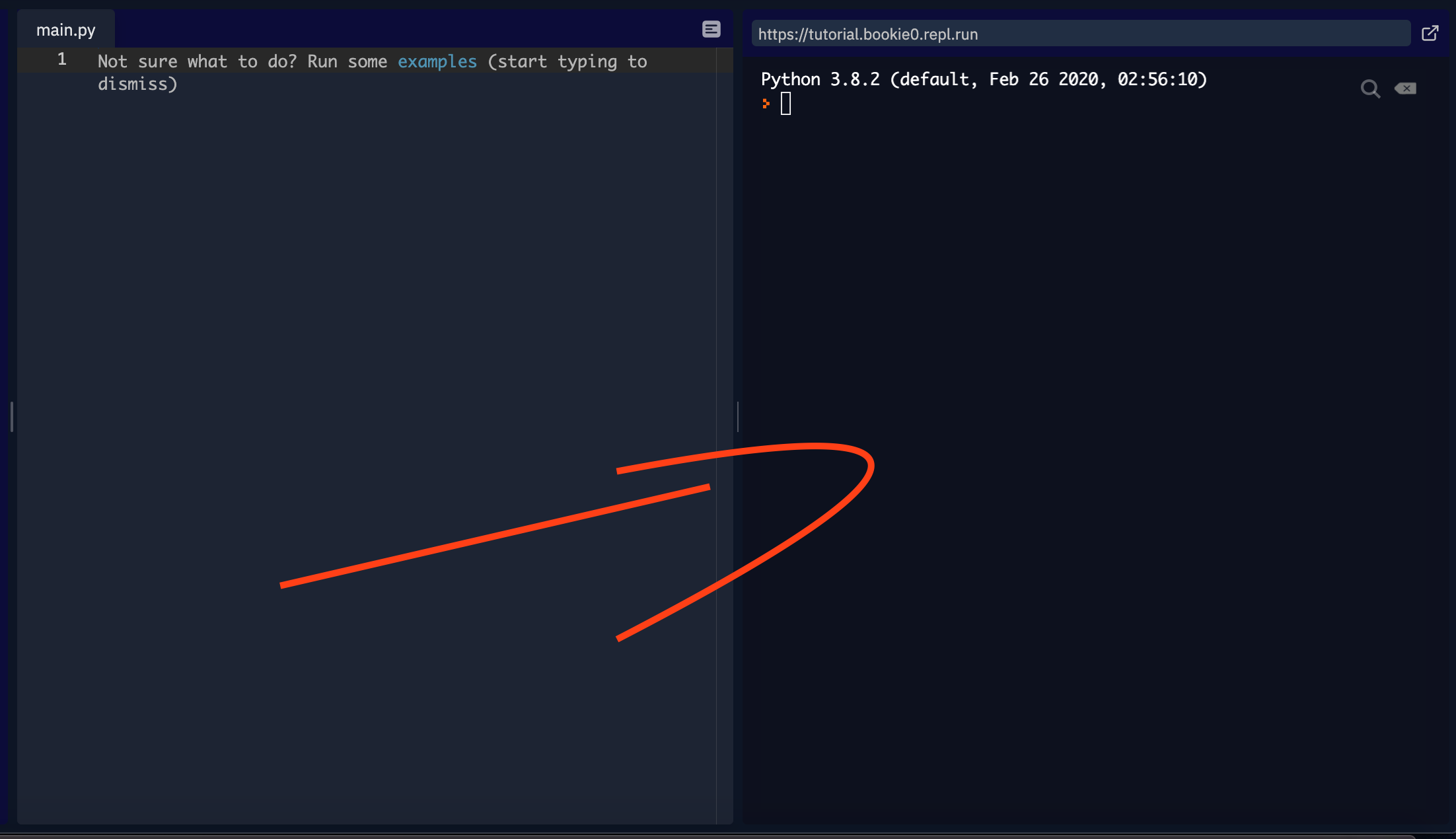• Input: stuff that is taken in by the computer (more on this later)

• Ouput: the information processed and sent out by the computer (usually in the console)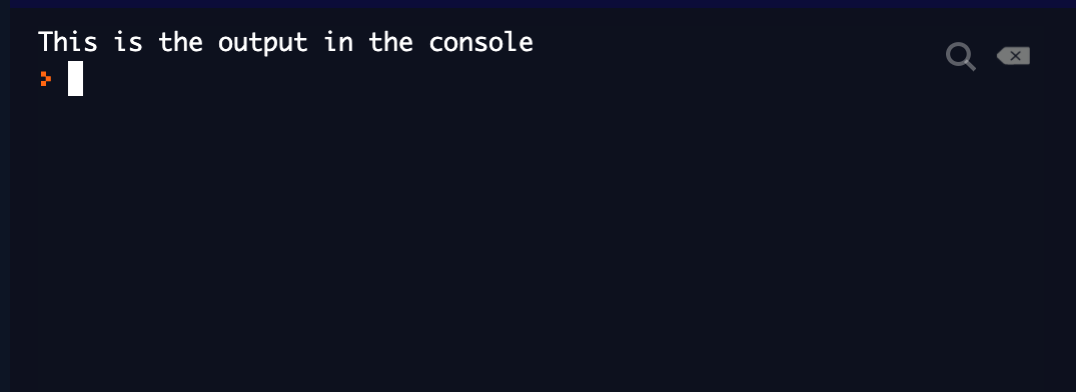• Errors: actually, a good thing! Don't worry if you have an error, just try to learn from it and correct it. That's how you can improve, by knowing how to correct errors.

• Execute: run a piece of code

Comments are used for explaining your code, making it more readable, and to prevent execution when testing code.

This is how to comment:

``````# this is a comment
# it starts with a hashtag #
# Python will ignore and not run anything after the hashtag ``````

You can also have multiline comments:

``````"""
this is
a multiline comment

I can make it very long!
"""``````

### print

The `print()` functions is used for outputting a message (object) onto the console. This is how you use it:

``````print("Something.")
# remember this is a comment
# you can use double quotes "
# or single quotes '
print('Using single quotes')
print("Is the same as using double quotes")``````

You can also triple quotes for big messages.

Example:

``````print("Hello World!")

print("""

Rules:

 Code
 Be nice
 Lol
 Repeat
""")``````

Output:

``````Hello World!

Rules:

 Code
 Be nice
 Lol
 Repeat ``````

### Data Types

Data types are the classification or categorization of data items.

These are the 4 main data types:

`int`: (integer) a whole number
12 is an `int`, so is 902.

`str`: (string) a sequence of characters
"Hi" is a `str`, so is "New York City".

`float`: (float) a decimal
-90 is a `float`, so is 128.84

`bool`: (boolean) data type with 2 possible values; `True` and `False`
Note that `True` has a capital `T` and `False` has a capital `F`!

### Variables

Variables are used for containing/storing information.

Example:

``````name = "Lucy"  # this variable contains a str

age = 25  # this variable contains an int

height = 160.5 # this variable contains a float

can_vote = True  # this variable contains a Boolean that is True (because Lucy is 25 y/o)``````

#### Printing variables:

To print variables, you simply do `print(variableName)`:

``````print(name)
print(age)
print(height)
print(can_vote)``````

Output:

``````Lucy
25
160.5
True``````

#### Naming Variables:

You should try to make variables with a descriptive name. For example, if you have a variable with an age, an appropriate name would be `age`, not `how_old` or `number_years`.

Some rules for naming variables:

• no spaces (use underscores)
• no keywords (like `print`, `input`, `or`, etc.)

#### Changing Variables:

You can change variables to other values.

For example:

``````x = 18
print(x)

x = 19
print(x)

# the output will be:
# 18
# 19``````

As you can see, we have changed the variable `x` from the initial value of 18 to 19.

### Concatenation

Let's go back to our first 3 variables:

``````name = "Lucy"

age = 25

height = 160.5 ``````

What if we want to make a sentence like this:
`Her name is Lucy, she is 25 years old and she measures 160.5 cm.`

Of course, we could just print that whole thing like this:
`print("Her name is Lucy, she is 25 years old and she measures 160.5 cm.")`

But if we want to do this with variables, we could do it something like this:

``````print("Her name is " + name + ", she is " + age + " years old and she measures " + height + " cm.")
# try running this!``````

Aha! If you ran it, you should have gotten this error: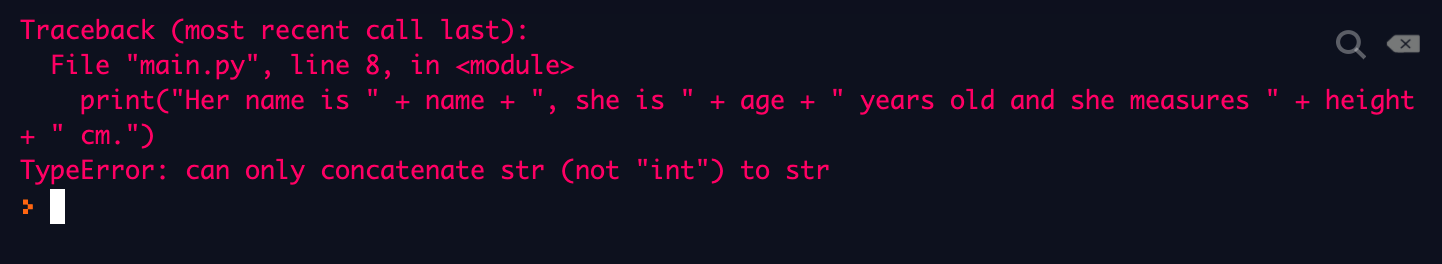Basically, it means that you cannot concatenate `int` to `str`. But what does concatenate mean?

Concatenate means join/link together, like the concatenation of "sand" and "castle" is "sandcastle"

In the previous code, we want to concatenate the bits of sentences ("Her name is ", ", she is", etc.) as well as the variables (`name`, `age`, and `height`).

Since the computer can only concatenate `str` together, we simply have to convert those variables into `str`, like so:

``````print("Her name is " + name + ", she is " + str(age) + " years old and she measures " + str(height) + " cm.")
# since name is already a str, no need to convert it``````

Output:

``Her name is Lucy, she is 25 years old and she measures 160.5 cm.``

### Operators

A symbol or function denoting an operation

Basically operators can be used in math.

List of operators:

• `+` For adding numbers (can also be used for concatenation) | Eg: 12 + 89 = 101
• `-` For subtracting numbers | Eg: 65 - 5 = 60
• `*` For multiplying numbers | Eg: 12 * 4 = 48
• `/` For dividing numbers | Eg: 60 / 5 = 12
• `**` Exponentiation ("to the power of") | Eg: 2**3 = 8
• `//` Floor division (divides numbers and takes away everything after the decimal point) | Eg: 100 // 3 = 33
• `%` Modulo (divides numbers and returns whats left (remainder)) | Eg: 50 % 30 = 20

These operators can be used for decreasing/increasing variables.

Example:

``````x = 12
x += 3
print(x)
# this will output 15, because 12 + 3 = 15``````

You can replace the `+` in `+=` by any other operator that you want:

``````x = 6
x *= 5
print(x)

y = 9
y /= 3
print(y)
# this will output 30 and then below 3.``````

Also: `x += y` is just a shorter version of writing `x = x + y`; both work the same

### Comparison Operators

Comparsion operators are for, well, comparing things. They return a Boolean value, `True` or `False`. They can be used in conditionals.

List of comparison operators:

• `==` equal to | Eg: 7 == 7
• `!=` not equal to | Eg: 7 != 8
• `>` bigger than | Eg: 12 > 8
• `<` smaller than | Eg: 7 < 9
• `>=` bigger than or equal to | Eg: 19 >= 19
• `<=` smaller than or equal to | Eg: 1 <= 4

If we type these into the console, we will get either `True` or `False`:

``````6 > 7 # will return False
12 < 80 # will return True
786 != 787 # will return True
95 <= 96 # will return True``````

### Conditionals

Conditionals are used to verify if an expression is `True` or `False`.

#### `if`

Example: we want to see if a number is bigger than another one.

How to say in english: "If the number 10 is bigger than the number 5, then etc.

How to say it in Python:

``````if 10 > 5:
# etc.``````

All the code that is indented will be inside that `if` statement. It will only run if the condition is verified.
You can also use variables in conditionals:

``````x = 20
y = 40

if x < y:
print("20 is smaller than 40"!)

# the output of this program will be "20 is smaller than 40"! because the condition (x < y) is True.``````

#### `elif`

`elif` is basically like `if`; it checks if several conditions are `True`

Example:

``````age = 16

if age == 12:
print("You're 12 years old!")

elif age == 14:
print("You're 14 years old!")

elif age == 16:
print("You're 16 years old!")``````

This program will output:

``You're 16 years old!``

Because `age = 16`.

#### `else`

`else` usually comes after the `if`/`elif`. Like the name implies, the code inside it only executes if the previous conditions are `False`.

Example:

``````age = 12

if age >= 18:
print("You can vote!")

else:
print("You can't vote yet!)``````

Output:

``You can't vote yet!``

Because `age < 18`.

### `input`

The `input` function is used to prompt the user. It will stop the program until the user types something and presses the return key.
You can assign the input to a variable to store what the user types.

For example:

``````username = input("Enter your username: ")

# then you can print the username

Output:

``````Enter your username: Bookie0
Welcome, Bookie0!``````

By default, the `input` converts what the user writes into `str`, but you can specify it like this:

``````number = int(input("Enter a number: ")) # converts what the user says into an int
# if the user types a str or float, then there will be an error message.

# doing int(input()) is useful for calculations, now we can do this:

number += 10
print("If you add 10 to that number, you get: "+ str(number)) # remember to convert it to str for concatenation!``````

Output:

``````Enter a number: 189
If you add 10 to that number, you get: 199``````

You can also do `float(input(""))` to convert it to `float`.

Now, here is a little program summarizing a bit of what you've learnt so far.

Full program:

``````username = input("Username: ")

print("Welcome Admin! You are the best!")

else:

else:

Now a detailed version:

``````# inputs

# variables

# conditionals

print("Welcome Admin! You are the best!")  # a welcome message only to the admin

print("Error! Wrong password!")  # an error message appears

else:  # if the user enters something different than the admin username
print("Welcome, general user "+str(username)+"!")  # a welcome message only for general users``````

Output:

An option:

``````Username: Mr.ADMIN

Another option:

``````Username: Mr.ADMIN
Welcome Admin! You are the best!``````

Final option:

``````Username: Bob
Welcome, general user Bob!``````

### A bit of lists

A list is a collection which is ordered and changeable. They are written with square braquets: `[]`

``````meat = ["beef", "lamb", "chicken"]

print(meat)``````

Output:

``['beef', 'lamb', 'chicken']``

You can access specific items of the list with the index number. Now here is the kinda tricky part. Indexes start at 0, meaning that the first item of the list has an index of 0, the second item has an index of 1, the third item has an index of 2, etc.

``````meat = ["beef", "lamb", "chicken"]
# Index:  0       1         2      etc.

print(meat)  # will output "chicken" because it is at index 2``````

You can also use negative indexing: index -1 means the last item, index -2 means the second to last item, etc.

``````meat = ["beef", "lamb", "chicken"]
# Index:  -3       -2         -1     etc.

print(meat[-3])  # will output "beef" because it is at index -3``````

You can add items in the list using `append()`:

``````meat = ["beef", "lamb", "chicken"]

meat.append("pork")

print(meat)``````

Output:

``['beef', 'lamb', 'chicken', 'pork']``

"pork" will be added at the end of the list.

For removing items in the list, use `remove()`:

``````meat = ['beef', 'lamb', 'chicken']

meat.remove("lamb")

print(meat)``````

Output:

``['beef', 'chicken']``

You can also use `del` to remove items at a specific index:

``````meat = ['beef', 'lamb', 'chicken']

del meat

print(meat)``````

Output:

``['lamb', 'chicken']``

There are also many other things you can do with lists, check out this: https://www.w3schools.com/python/python_lists.asp for more info!

### `for` loops

A for loop is used for iterating over a sequence. Basically, it runs a piece of code for a specific number of times.

For example:

``````for i in range(5):
print("Hello!")``````

Output:

``````Hello!
Hello!
Hello!
Hello!
Hello!``````

You can also use the `for` loop to print each item in a list (using the list from above):

``````meat = ['beef', 'lamb', 'chicken']

for i in meat:
print(i)``````

Output:

``````beef
lamb
chicken``````

### `while` loops

`while` loops will run a piece of code as long as the condition is `True`.

For example:

``````x = 1  # sets x to 1

while x <= 10:  # will repeat 10 times
print(x)  # prints x
x += 1  # increments (adds 1) to x``````

Ouput:

``````1
2
3
4
5
6
7
8
9
10``````

You can also make `while` loops go on for infinity, like so (useful for spamming lol):

``````while True:
print("Theres no stopping me nowwwww!")``````

Output:

``````Theres no stopping me nowwwww!
Theres no stopping me nowwwww!
Theres no stopping me nowwwww!
Theres no stopping me nowwwww!
Theres no stopping me nowwwww!

# etc until infinity``````

### Functions

Functions are a group of code that will only execute when it is called.

For example, instead having to type a piece of code several times, you can use a function to put that piece of code inside, and then when you need to use it, you can just call it.

``````def greeting():  # defining the function
print("Bonjour!")  # everything that is indented will be executed when the function is called

greeting()  # calling the function
# you can now call this function when you want, instead of always writing the same code everytime``````

Output:

``Bonjour!``

#### return and arguments

The `return` statement is used in function. It ends the function and "returns" the result, i.e. the value of the expression following the return keyword, to the caller. It is not mandatory; you don't have to use it.

You can also have arguments inside a functions. This allows you to change the function values. The arguments are in the parenthesis.

For example:

``````def sum(x, y): # x and y are the arguments
total = x + y

result = sum(4, 5) # you can change those to what you want
print(result) # this will output 9, because 4+5 = 9``````

### Imports

#### `time`

You can use time in your Python programs.

How to make the program wait:

``````# first import time
import time

print("Hello!")

# then for the program to wait
time.sleep(1)  # write how long you want to wait (in seconds) in the parenthesis

print("Bye!")``````

Output:

``````Hello!
# program will wait 1 second
Bye!``````

You can also do this (more simpler):

``````import time
from time import sleep

# instead of time.sleep(), do sleep()
# its the same

print("time.sleep(1)...")

time.sleep(1)

print("...is the same as...")

sleep(1)

print("sleep(1)!")``````

#### `random`

You can use the `random` module to randomly pick numbers with `randint()`:

``````# remember to import!
import random
from random import randint

rand_num = randint(1,5)
# this will output a random number between 1 and 5 inclusive!
# this means the possible numbers are 1, 2, 3, 4, or 5``````

The reason I am precising this is because you can also use `randrange()`:

``````import random
from random import randrange

rand_num = randrange(1,5)
# this will output a random number between 1 inclusive and 5 NON-inclusive (or 4 inclusive)!
# this means the possible numbers are 1, 2, 3, or 4``````

You can also randomly pick an item from a list with `choice()`:

``````import random
from random import choice

meat = ["beef", "lamb", "chicken"]
rand_meat = choice(meat)

print(rand_meat)

# this will output a randomly chosen item of the list meat
# the possible outcomes are beef, lamb, or chicken.``````

#### `math`

First, you already have some functions already built in Python: `min()` and `max()`. They return the smallest and biggest value of `ints` inside the parenthesis, respectively.

For example:

``````list_a = min(18, 12, 14, 16)
list_b = max(17, 19, 15, 13)

print(list_a)  # will output 12
print(list_b)  # will output 19``````

Now for some more modules:

You can use `math.floor()` and `math.ceil()` to round up numbers to the nearest or highest `int`.

For example:

``````# first import
import math

num_a = math.floor(2.3)
num_b = math.ceil(2.3)

print(num_a)  # will output 2
print(num_b)  # will output 3``````

Explanation (from Andrew Sutherland's course): So `math.floor()` will round up `2.3` to the nearest lowest `int`, which in this case is `2`. This is because, if you imagine it, the floor is on the bottom, so thats why it will round the number to the nearest lowest `int`.

Vice-versa for `math.ceil()`; it will round up `2.3` to the nearest highest `int`, which in this case is `3`. This is because `ceil` is short for ceiling (programmers like to shorten words), and the ceiling is high.

You can also get `pi` π:

``````import math

pi = math.pi

print(pi)  ``````

Output:

``3.141592653589793``

Here is the full list of all the things you can do with `math`: https://www.w3schools.com/python/module_math.asp

### Small Programs You Can Use

#### Countdown Program:

``````# imports
import time
from time import sleep

def countdown():  # making a function for the countdown (so you can use it several times)

count = int(input("Countdown from what? "))  # asks user how long the countdown

while count >= 0:  # will repeat until count = 0
print(count)  # prints where the countdown is at
count -= 1  # subtracts 1 from count
sleep(1)  # program waits 1 second before continuing

print("End of countdown!")  # message after the countdown

countdown()  # remember to call the function or nothing will happen``````

Output:

``````Countdown from what? 5
5
4
3
2
1
0
End of countdown!``````

#### Simple Calculator

First way using `eval()`

``````calculation = input("Type your calculation: ")  # asks the user for a calculation.

print("Answer to " + str(calculation) + ": " + str(eval(calculation)))
# eval basically does the operation, like on a normal calculator.

# however, if you write something different than a valid operaion, there will be an error.``````

Or another way, using several conditionals, and you can only do "something" + "something" (but with the operators):

``````def calculator():  # making a function to hold all the code for calculator

while True:  # loops forever so you can make several calculations without having to press run again

first_num = int(input("Enter 1st number: "))  # asks user for 1st number

second_num = int(input("Enter 2nd number: "))  # asks user for 2nd number
operator = input("Select operator: +  -  *  /  **  //  ")  # asks user for operator

if operator == "+":  # addition

elif operator == "-":  # subtraction

elif operator == "*":  # multiplication

elif operator == "/":  # division

elif operator == "**":  # exponentiation ("to the power of")

elif operator == "//":  # floor division

else:  # if user selects an invalid operator
print("Invalid!")

calculator()  # calls the function ``````

But obviously that is pretty long and full of many `if`/`elif`.

Some functions that are useful:

"Press ENTER to continue" Prompt:

``````def enter():
input("Press ENTER to continue! ")

# this is useful for text based adventure games; when they finish reading some text, they can press ENTER and the next part will follow.
# just call the function where you need it``````

Spacing in between lines function:

``````def space():
print()
print()

# same as pressing ENTER twice, this is useful to make your text a bit more airy, makes it less compact and block like.``````

Slowprint:

``````# first imports:
import time, sys
from time import sleep

def sp(str):
for letter in str:
sys.stdout.write(letter)
sys.stdout.flush()
time.sleep(0.06)
print()

# to use it:

sp("Hello there!")

# this will output Hello There! one letter every 0.06 seconds, making it look like the typewriter effect.``````

### `ANSI` Escape Codes

`ANSI` escape codes are for controlling text in the console. You can use it to make what is in the output nicer for the user.

For example, you can use `\n` for a new line:

``name = input("Enter your name\n>>> ")``

Output:

``````Enter your name
>>> ``````

This makes it look nice, you can start typing on the little prompt arrows `>>>`.

You can also use `\t` for tab:

``print("Hello\tdude")``

Output:

``Hello   dude``

`\v` for vertical tab:

``print("Hello\vdude")``

Output:

``````Hello
dude``````

You can also have colors in python:

``````# the ANSI codes are stored in variables, making them easier to use
black = "\033[0;30m"
red = "\033[0;31m"
green = "\033[0;32m"
yellow = "\033[0;33m"
blue = "\033[0;34m"
magenta = "\033[0;35m"
cyan = "\033[0;36m"
white = "\033[0;37m"
bright_black = "\033[0;90m"
bright_red = "\033[0;91m"
bright_green = "\033[0;92m"
bright_yellow = "\033[0;93m"
bright_blue = "\033[0;94m"
bright_magenta = "\033[0;95m"
bright_cyan = "\033[0;96m"
bright_white = "\033[0;97m"

# to use them:
print(red+"Hello")

# you can also have multiple colors:
print(red+"Hel"+bright_blue+"lo")

# and you can even use it with the slowPrint I mentioned earlier!``````

Output: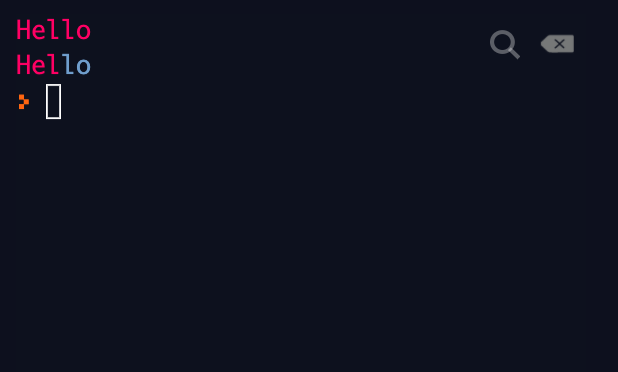And you can have underline and italic:

``````reset = "\u001b[0m"
underline = "\033[4m"
italic = "\033[3m"

# to use it:

print(italic+"Hello "+reset+" there "+underline+"Mister!")
# the reset is for taking away all changes you've made to the text
# it makes the text back to the default color and text decorations.``````

Output: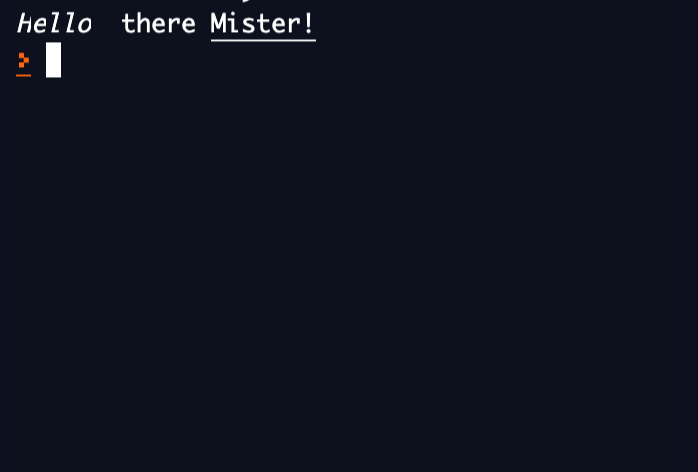### Links: Sources and Good Websites

#### Sources:

Always good to use a bit of help from here and there!

Interactive:

### `Goodbye World!`: End

Well, I guess this is the end. I hope y'all have learnt something new/interesting! If you have any questions, please comment and I will try my best to answer them.

# Have a super day everyone!

###### My beautiful `ASCII` art: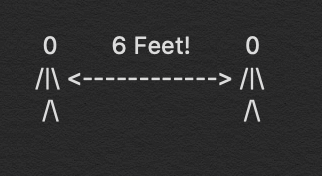You are viewing a single comment. View All
firefish (885)

`\e[38;5;166m`Great tutorial! ANSI codes aren't part of python... they are... part of the terminal`\e[0m`

Bookie0 (5061)

oh ok lol but i still put them because they are useful to know @firefish

firefish (885)

@Bookie0 Well you shouldn't say tthat they are exclusive to python

Bookie0 (5061)

i didnt...did i? i just said ANSI escape codes as the title, but yes you're right you can use them in other langs too @firefish